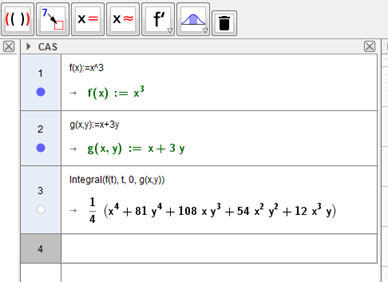# Integral and surface

Elyesse shared this question 1 year ago

Hello,

First i apologize for my poor english.

I would like to know how to have the surface of a function like:

z(x,y)=integral(f(t),0,g(x,y))

The problem is that geogebra always take x as integral variable, i would like to change it in t.

Then i would like to change the limit of integration to a variable of x and y, in order to have the surface of it.

Thanks1

hello

use the CAS view1

Hi, Unfortunately, for each point (x, y) of the space the integral is not numerically counted as, for example, in http://mathworld.wolfram.co....